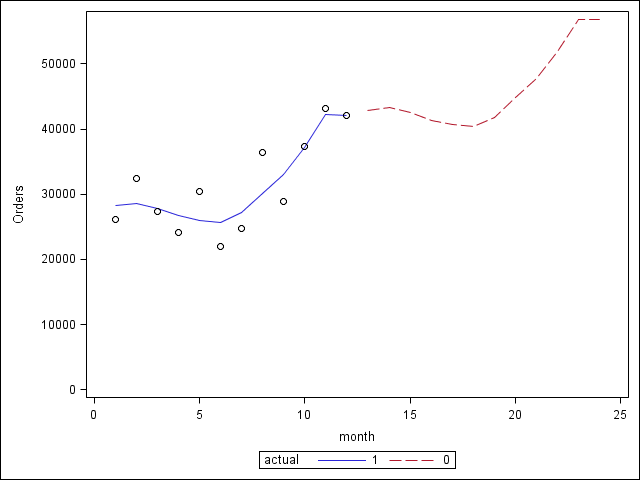## Polynomial equation for sales forecast

Hi Experts,

I've 12 months data (Number of orders) and trying to explore a model which can be used for forecasting. I found 2nd degree polynomial model which seems to represent pattern in a better way.  Value of R²=0.70. Please see below the equation.

 y = 308.77x2 - 2662.8x + 31837

Can we use polynomial equation for sales forecast? My concern is when we go further into fututre orders per month will soon be doubled which business people will not accept. Any suggestions on this one please. I've attached chart for your review please.

Regards,

1 ACCEPTED SOLUTION

Accepted SolutionsPGStats
Opal | Level 21

## Re: Polynomial equation for sales forecast

I agree with Paige Miller. Fitting a quadratic curve to a time series is perilous. With twelve points, you will necessarily have to make many assumptions to build any kind of predictive model. For example

1) There is a trend that will go on next year

2) There is a seasonal pattern that will repeat next year

data test;

infile datalines missover;

input month Orders;

yearMonth = mod(month,12);

actual= not missing(orders);

datalines;

1 26156

2 32396

3 27415

4 24129

5 30350

6 21992

7 24705

8 36434

9 28858

10 37353

11 43137

12 42119

13

14

15

16

17

18

19

20

21

22

23

24

;

title;

proc gam data=test;

model orders = param(month) spline(yearmonth);

output out=gamout predicted;

run;

proc sgplot data=gamout;

series x=month y=p_orders/ group=actual;

scatter x=month y=orders;

yaxis min=0;

run;PG
6 REPLIES 6

## Re: Polynomial equation for sales forecast

Hard to say, without more VERY BASIC information

What is x?

What is y?

--
Paige Miller

## Re: Polynomial equation for sales forecast

Hi Paige,

x is month (From 1 to 12)

y is number of orders

Please see below table that contains month, orders and predicted orders. At month 20 predicted orders become almost 100,000.

Regards,

 y = 308.77x2 - 2662.8x + 31837 month Orders Predicted Orders 1 26156 29482.97 2 32396 27746.48 3 27415 26627.53 4 24129 26126.12 5 30350 26242.25 6 21992 26975.92 7 24705 28327.13 8 36434 30295.88 9 28858 32882.17 10 37353 36086 11 43137 39907.37 12 42119 44346.28 13 49402.73 14 55076.72 15 61368.25 16 68277.32 17 75803.93 18 83948.08 19 92709.77 20 102089

## Re: Polynomial equation for sales forecast

The idea that month number has a quadratic relationship with orders ... well, let me just say that this is bizarre ... I wouldn't use such a model, even if the R-squared was 0.7. You have discovered a coincidence, not a predictive model.

Normally, forecasts of economic activity ... in this case orders ... are based upon time series models, perhaps with some seasonality built in, with X being some measures of customer's economic well being, or the economic well being of the economy as whole (or both)

--
Paige Millerudo_sas
SAS Employee

## Re: Polynomial equation for sales forecast

Hello -

This might be a little bit off topic, but R square should not be used for time series forecasts. For one thing, it overlooks bias in forecasts. A model can have a perfect R square, yet the values of the forecasts could be substantially different from the values for all forecasts. Also, a model could have an R square of zero but provide perfect forecasts if the mean were forecasted correctly and no variation occurred in the data.

Thanks,

UdoPGStats
Opal | Level 21

## Re: Polynomial equation for sales forecast

I agree with Paige Miller. Fitting a quadratic curve to a time series is perilous. With twelve points, you will necessarily have to make many assumptions to build any kind of predictive model. For example

1) There is a trend that will go on next year

2) There is a seasonal pattern that will repeat next year

data test;

infile datalines missover;

input month Orders;

yearMonth = mod(month,12);

actual= not missing(orders);

datalines;

1 26156

2 32396

3 27415

4 24129

5 30350

6 21992

7 24705

8 36434

9 28858

10 37353

11 43137

12 42119

13

14

15

16

17

18

19

20

21

22

23

24

;

title;

proc gam data=test;

model orders = param(month) spline(yearmonth);

output out=gamout predicted;

run;

proc sgplot data=gamout;

series x=month y=p_orders/ group=actual;

scatter x=month y=orders;

yaxis min=0;

run;PG

## Re: Polynomial equation for sales forecast

Thanks PG for suggesting a solution.

Discussion stats
• 6 replies
• 3648 views
• 9 likes
• 4 in conversation